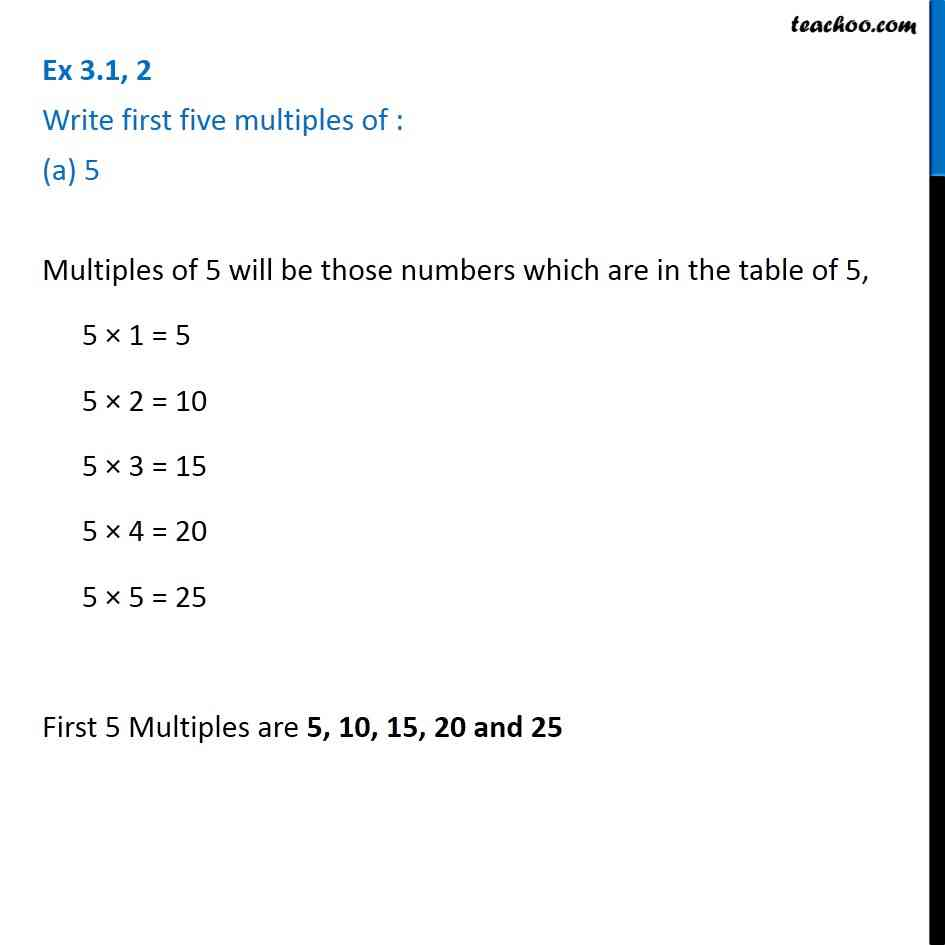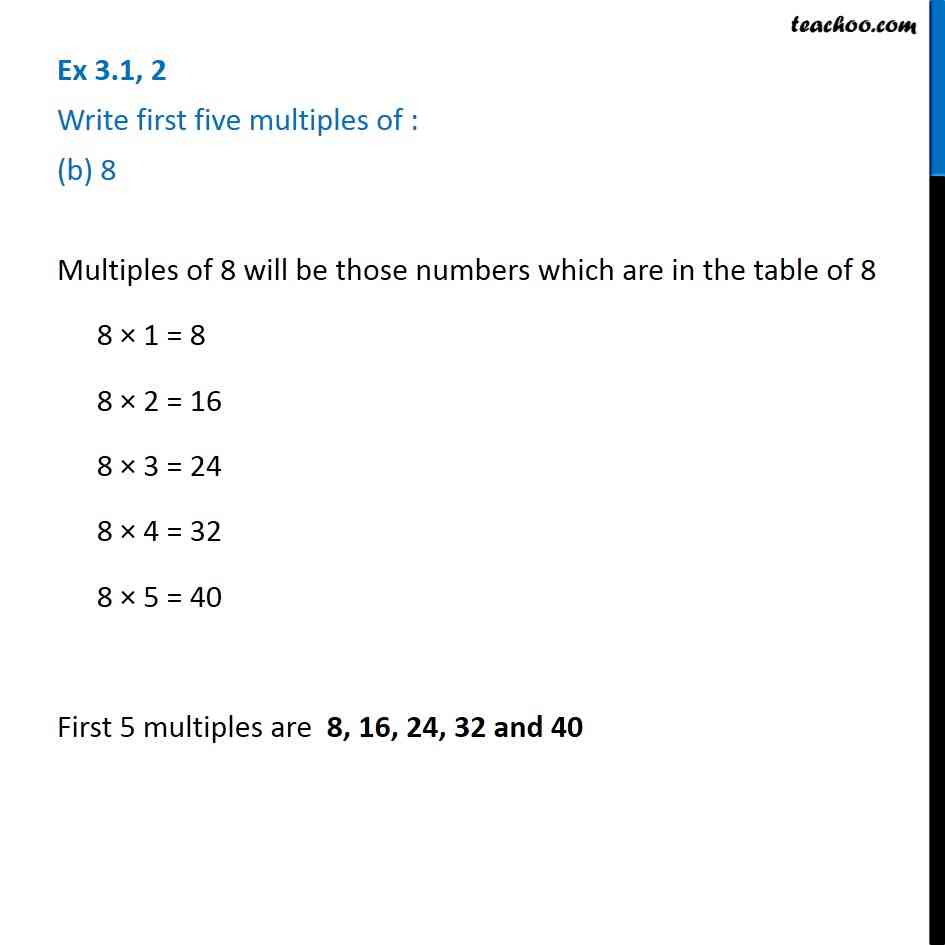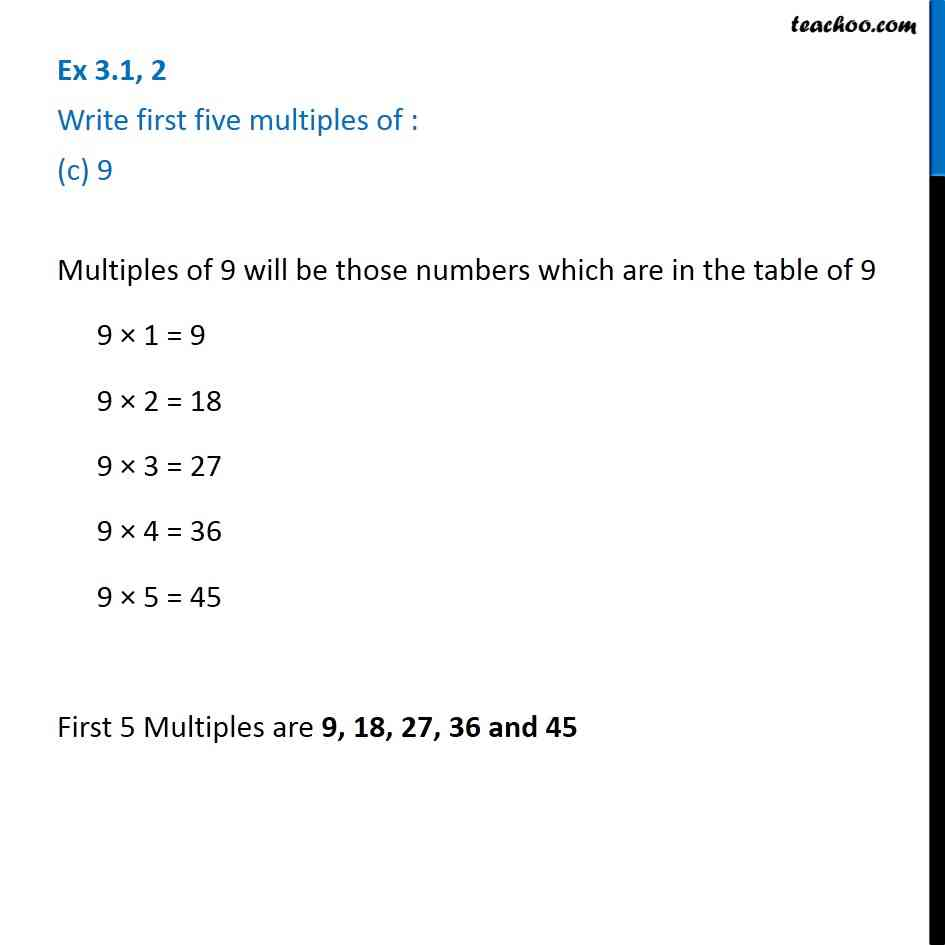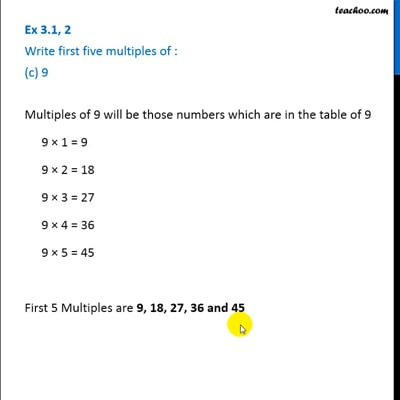Ex 3.1

Chapter 3 Class 6 Playing with Numbers
Serial order wiseThis video is only available for Teachoo black users

Introducing your new favourite teacher - Teachoo Black, at only ₹83 per month

### Transcript

Ex 3.1, 2 Write first five multiples of : (a) 5 Multiples of 5 will be those numbers which are in the table of 5, 5 1 = 5 5 2 = 10 5 3 = 15 5 4 = 20 5 5 = 25 First 5 Multiples are 5, 10, 15, 20 and 25 Ex 3.1, 2 Write first five multiples of : (b) 8 Multiples of 8 will be those numbers which are in the table of 8 8 1 = 8 8 2 = 16 8 3 = 24 8 4 = 32 8 5 = 40 First 5 multiples are 8, 16, 24, 32 and 40 Ex 3.1, 2 Write first five multiples of : (c) 9 Multiples of 9 will be those numbers which are in the table of 9 9 1 = 9 9 2 = 18 9 3 = 27 9 4 = 36 9 5 = 45 First 5 Multiples are 9, 18, 27, 36 and 45